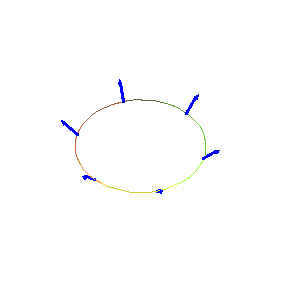Function: curvarrows - plot a 2D or 3D curve (parametric or cartesian) with arrows from a vector field at points on the curve.

Calling sequence:

curvarrows(fld,curv,t=a..b);
Parameters:

fld - the vector field, either a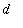-component list or vector expression in the parameter t (specifying the vectors in terms of the parameter) or a function ofvariables whose values are-component lists or vectors (specifying the vector field in terms of Cartesian coordinates), whereis 2 or 3.

curv - the curve, either a-component list or vector expression in the parameter t (for a parametric representation of the curve) or a scalar expression in t (representing the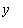coordinate while t is the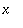coordinate).

t - name for the parameter.

a , b - real constants, endpoints for the parameter interval.

Optional arguments:

arrownum=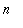forarrows along the curve, equally spaced in terms of the parameter (default is arrownum=10 )

scalefactor=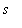to multiply arrow lengths by real constant.

arrowthickness=for thickness of the arrow shaft (should be 0, 1, 2 or 3, default is the same as the thickness option if specified, or 3 if not).

arrowcolour=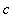to specify arrow colour (default is the same as the colour option if specified, or red if not). The alternate spelling arrowcolor is allowed.

other 2D or 3D curve plotting options

Description:

This function creates a 2D or 3D plot data object which consists of a curve specified by curv for t = a .. b (including all options of the curvarrows command except arrownum , scalefactor , arrowthickness and arrowcolour ), plus arrows representing the vector field fld drawn at points on the curve.

The curve is drawn using plot (in either the parametric or Cartesian form) for the 2D case, or spacecurve for the 3D case.

Unless the option scaling=constrained ("Projection, Constrained" on the plot menu or "1-1" on the context bar) is used, the shapes of the arrowheads may be distorted.

This function is part of the package surfarro in the Maple Advisor Database library, and must be loaded before use by the command with(surfarro); or with(surfarro,curvarrows);

Examples:

> with(surfarro,curvarrows):

Curve is a circle represented parametrically, vectors are tangent.

> curv1:= [cos(t),sin(t)];
F1:= diff(curv1,t);
curvarrows(F1, curv1, t = 0 .. 2*Pi, arrowcolour=blue,
scalefactor=1/2, arrowthickness=1, scaling=constrained);

`                               curv1 := [cos(t), sin(t)]`

`                                F1 := [-sin(t), cos(t)]`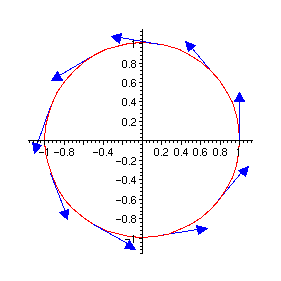Curve is a semicircle represented in Cartesian coordinates, vectors are normal.

> curv2:= sqrt(1-x^2);
F2:= [x,curv2];
curvarrows(F2,curv2, x = -1 .. 1,
scalefactor=1/3, scaling=constrained);

```                                                    2
curv2 := sqrt(1 - x )```

```                                                    2
F2 := [x, sqrt(1 - x )]```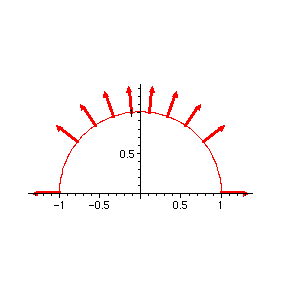Parametric 3D curve, vector field is a function of,and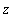coordinates. Note that 6 arrows appear because the arrows at the beginning and end of the parameter interval coincide.

> curv3:= [cos(t), sin(t), 1];
F3:= (x,y,z) -> [x, y, z];
curvarrows(F3,curv3, t = 0 .. 2*Pi,
arrowcolour=blue, scalefactor = 1/4, arrownum = 7, scaling=constrained);

`                              curv3 := [cos(t), sin(t), 1]`

`                              F3 := (x, y, z) -> [x, y, z]`